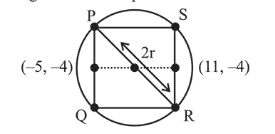# A square is inscribed in the circle

Question:

A square is inscribed in the circle $x^{2}+y^{2}-6 x+8 y-103=0$ with its sides parallel to the coordinate axes. Then the distance of the vertex of this square which is nearest to the origin is :

1. (1) 6

2. (2) $\sqrt{137}$

3. (3) $\sqrt{41}$

4. (4) 13

Correct Option: , 3

Solution:

The equation of circle is,

$x^{2}+y^{2}-6 x+8 y-103=0$

$\Rightarrow \quad(x-3)^{2}+(y+4)^{2}=(8 \sqrt{2})^{2}$

$C(3,-4), r=8 \sqrt{2}$

$\Rightarrow \quad$ Length of side of square $=\sqrt{2} r=16$$\Rightarrow \quad P(-5,4), Q(-5,-12)$

$R(11,-12), S(11,4)$

$\Rightarrow \quad$ Required distance $=O P$

$=\sqrt{(-5-0)^{2}+(-4-0)^{2}}=\sqrt{25+16}=\sqrt{41}$